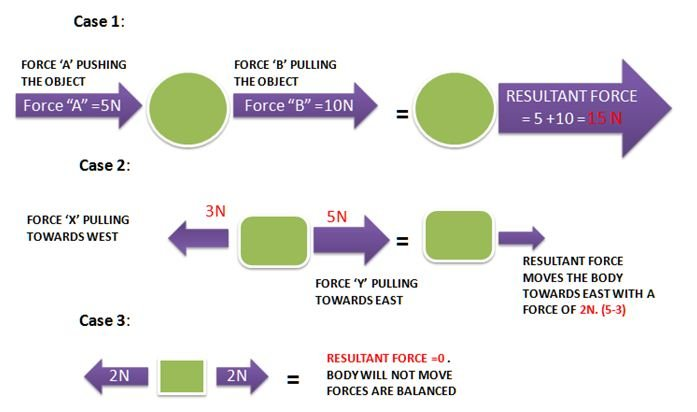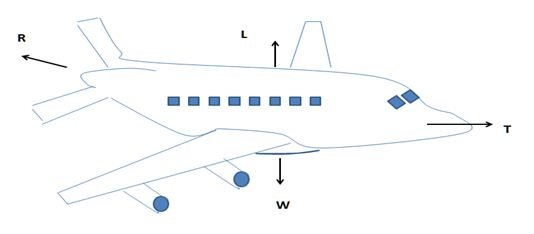# Definition Of Resultant Force

## Definition Of Resultant Force

When two or more forces acting on an object in a straight line then we can add or subtract to get the resultant force on the object.#### Example :

In The Above Diagram
Case 1: Two forces are acting in same direction, hence to get the resultant force we add the respective forces.
Case 2: Two forces are acting in opposite direction; hence the resultant force is obtained by subtracting the respective forces. Resultant force will act towards the direction of large force.
Case 3: When the forces are balanced (Equal forces are acting in opposite direction to each other), the Vertical forces | Horizontal forces resultant force will be equal to zero.

### More About Resultant Force :

If the resultant force acting on stationary object is not zero, then the body moved in the direction of the resultant force.
Resultant force acting on a body gives the direction of motion of a body.
Example: An aircraft is flying at constant height and gaining speed.
Four forces are acting on the air craft in four different directions.
L- Lift due to wings
R- Air resistance
W- The weight of the air-craft
T- The thrust due to the engines.Which of the following is correct?

 A L = W T = R B L > W T > R C L� = W T > R D L > W T = R